## Mathematical-Reasoning

 Question 1
Which is the number that comes next in the following sequence?
2, 6, 12, 20, 30, 42, 56, _____
 A 60 B 64 C 72 D 70
Mathematical-Reasoning       Series-Test       2012 June UGC NET Paper 1
Question 1 Explanation:Question 2
Find the next letter for the series YVSP .........
 A N B M C O D L
Mathematical-Reasoning       Alphabet-Test       2012 June UGC NET Paper 1
Question 2 Explanation: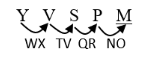Question 3
Given that in a code language, ‘645’ means ‘day is warm’; ‘42’ means ‘warm spring’ and ‘634’ means ‘spring is sunny’; which digit represents ‘sunny’ ?
 A 3 B 2 C 4 D 5
Mathematical-Reasoning       Coding-and-Decoding       2012 June UGC NET Paper 1
Question 3 Explanation:
645 = Day is warm
42 = Warm spring
634 = Spring is sunny
From the option 2 is not possible. 5 also not possible.
4 is present in first statement.
Only ‘3’ is possible.
 Question 4
The basis of the following classification is :
‘first President of India’ ‘author of Godan’ ‘books in my library’, ‘blue things’ and ‘students who work hard’
 A Common names B Proper names C Descriptive phrases D Indefinite description
Mathematical-Reasoning       Classification       2012 June UGC NET Paper 1
 Question 5
Six persons A, B, C, D, E and F are standing in a circle. B is between F and C, A is between E and D, F is to the left of D. Who is between A and F ?
 A B B C C D D E
Mathematical-Reasoning       Seating-Arrangement       2012 June UGC NET Paper 1
 Question 6
The price of petrol increases by 25%. By what percentage must a customer reduce the consumption so that the earlier bill on the petrol does not alter ?
 A 20% B 25% C 30% D 33.33%
Mathematical-Reasoning       Percentage       2012 June UGC NET Paper 1
Question 6 Explanation:
Let cost of petrol = Rs. 100 Say
The cost is increased by 25% then cost of petrol = Rs 125
Consumption should reduced =[(25%/125) * 100]=20%
 Question 7
If Ram knows that y is an integer greater than 2 and less than 7 and Hari knows that y is an integer greater than 5 and less than 10, then they may correctly conclude that
 A y can be exactly determined B y may be either of two values C y may be any of three values D there is no value of y satisfying these conditions
Mathematical-Reasoning       Number-Theory       2012 June UGC NET Paper 1
 Question 8
Four pipes can fill a reservoir in 15, 20, 30 and 60 hours respectively. The first one was opened at 6 AM, second at 7 AM, third at 8 AM and the fourth at 9 AM. When will the reservoir be filled ?
 A 11 AM B 12 Noon C 1 PM D 1:30 PM
Mathematical-Reasoning       Distance-and-Time       2012 June UGC NET Paper 1
 Question 9
Choose the proper alternative given in the codes to replace the question mark
Bee-Honey, Cow-Milk, Teacher-?
 A Intelligence B Marks C Lessons D Wisdom
Mathematical-Reasoning       Analogy-Test       2013 June UGC NET Paper 1
 Question 10
P is the father of R and S is the son of Q and T is the brother of P. If R is the sister of S, how is Q related to T
 A Wife B Sister-in-law C Brother-in-law D Daughter-in-law
Mathematical-Reasoning       Blood-Relation-Test       2013 June UGC NET Paper 1
 Question 11
In a certain code GALIB is represented by HBMJC. TIGER will be represented by
 A UJHFS B UHJSE C JHUSF D HUJSE
Mathematical-Reasoning       Coding-and-Decoding       2013 June UGC NET Paper 1
Question 11 Explanation:Question 12
In a certain cricket tournament 45 matches were played. Each team played once against each of the other teams. The number of teams participated in the tournament is
 A 8 B 10 C 12 D 210
Mathematical-Reasoning       Averages       2013 June UGC NET Paper 1
Question 12 Explanation:Question 13
The missing number in the series
40, 120, 60, 180, 90, ?, 135 is
 A 110 B 270 C 105 D 210
Mathematical-Reasoning       Series-Test       2013 June UGC NET Paper 1
Question 13 Explanation:
40, 120, 60, 180, 90, 270, 135
40 * 3 = 120
60 * 3 = 180
90 * 3 = 270
 Question 14
The odd numbers from 1 to 45 which are exactly divisible by 3 are arranged in an ascending order. The number at 6 th position is
 A 18 B 24 C 33 D 36
Mathematical-Reasoning       Number-Theory       2013 June UGC NET Paper 1
 Question 15
The mean of four numbers a,b,c,d is 100. IF c = 70, then the mean of the remaining numbers is
 A 30 B 85/2 C 170/3 D 110
Mathematical-Reasoning       Averages       2013 June UGC NET Paper 1
Question 15 Explanation:Question 16
If the radius of a circle is increased by 50%, the perimeter of the circle will increased by
 A 20% B 30% C 40% D 50%
Mathematical-Reasoning       Geometry       2013 June UGC NET Paper 1
 Question 17
The next term in the series: AB, ED, IH, NM, --- is
 A TS B ST C TU D SU
Mathematical-Reasoning       Alphabet-Test       2014 December UGC NET Paper 1
Question 17 Explanation:Question 18
If STREAMERS is coded as UVTGALDQR, then KNOWLEDGE will be coded as
 A MQPYLCDFD B MPQYLDCFD C PMYQLDFCD D YMQPLDDFC
Mathematical-Reasoning       Coding-and-Decoding       2014 December UGC NET Paper 1
Question 18 Explanation:Question 19
A is brother of B. B is the brother of C. C is the husband of D. E is the father of A. D is related to E as
 A Daughter B Daughter-in-law C Sister-in-law D Sister
Mathematical-Reasoning       Blood-Relation-Test       2014 December UGC NET Paper 1
Question 19 Explanation:
A, B, C are Brothers.
C, D are Couple.
E is father of A then E is father to A, B, C.
E is father to C, then D is daughter-in-law to E.
 Question 20
Two numbers are in the ration 3:5. If 9 is subtracted from the numbers, the ratio becomes 12:23. The numbers are
 A 30, 50 B 36, 60 C 33, 55 D 42, 70
Mathematical-Reasoning       Ratio-and-Proportion       2014 December UGC NET Paper 1
Question 20 Explanation:
A : B = 3 : 5 → (1)
(A - 9) : (B - 9) = 12 : 23 → (2)
Substitute values 33, 55 then it satisfies equations (1) & (2).
Ans:- C
 Question 21
The mean of the ages of father and his son is 27 years. After 18 years, father will be twice as old as his son. Their present ages are
 A 42, 12 B 40, 14 C 30, 24 D 36, 18
Mathematical-Reasoning       Age-Related-Test       2014 December UGC NET Paper 1
 Question 22
The next term in the series: 2, 7, 28, 63, 126, --- is
 A 215 B 245 C 276 D 296
Mathematical-Reasoning       Series-Test       2014 December UGC NET Paper 1
Question 22 Explanation:
2, 7, 28, 63, 126, 215
13+1, 23-1, 33+1, 43-1, 53+1, 63-1
 Question 23
In certain code TEACHER is written as VGCEJGT. The code of CHILDREN will be
 A EKNJFTGP B EJKNFTGP C KNJFGTP D None of these
Mathematical-Reasoning       Coding-and-Decoding       2013 December UGC NET Paper 1
Question 23 Explanation:Question 24
A person has to buy both apples and mangoes. The cost of one apple is Rs 7/- whereas that of mango is Rs 5/-
If the person has Rs. 38, the number of apples he can buy is
 A 1 B 2 C 3 D 4
Mathematical-Reasoning       Averages       2013 December UGC NET Paper 1
Question 24 Explanation:
The cost of apple = 7
The cost of mango = 5
The person has 38 Rupees = (7 * 4)+(5*2) = 38
∴ The person can be able to buy 4 apples.
 Question 25
A man pointing to a lady said, "The son of her only brother is the brother of my wife," The lady is related to the man as
 A Mother's sister B Grand mother C Mother-in-law D Sister of Father-in-law
Mathematical-Reasoning       Blood-Relation-Test       2013 December UGC NET Paper 1
Question 25 Explanation:
Brother of my wife - My brother-in-law.
Son of lady’s brother is the brother-in-law of the man. So lady’s brother is man’s father-in-law.
Then the lady is the sister of man’s father-in-law.
 Question 26
In this series 6, 4, 1,2,2,8, 7,4,2,1,5,3,8,6,2,2,7,1,4,1,3,5,8,6.
How many pairs of successive numbers have a difference of 2 each?
 A 4 B 5 C 6 D 8
Mathematical-Reasoning       Series-Test       2013 December UGC NET Paper 1
Question 26 Explanation:
6, 4, 1, 2, 2, 8, 7, 4, 2, 1, 5, 3, 8, 6, 2, 2, 7, 1, 4, 1, 3, 5, 8, 6
⇒(6,4), (4,2), (5,3), (8,6), (8,6)
Ans:- B
 Question 27
The mean marks obtained by a class of 40 students is 65; The mean marks of half of the students is found to be 45. The mean marks of the remaining students is
 A 85 B 60 C 70 D 65
Mathematical-Reasoning       Averages       2013 December UGC NET Paper 1
Question 27 Explanation:Question 28
Anil is twice as old as Sunita. Three years ago, he was three times as old as Sunita. The present age of Anil is
 A 6 years B 8 years C 12 years D 16 years
Mathematical-Reasoning       Age-Related-Test       2013 December UGC NET Paper 1
 Question 29
The world population growth rate at a certain reference year was 3.5%. Assuming exponential growth of population, after how many years, the population of the world would have increased by a factor 16 ?
 A ~ 80 years B ~ 40 years C ~ 160 years D ~ 320 years
Mathematical-Reasoning       Growth-Rate       2013 September UGC NET Paper 1
 Question 30
A person writes all the numbers from 0 to 99. The number of times digit 3 will be written is
 A 18 B 19 C 20 D 21
Mathematical-Reasoning       Number-Theory       2013 September UGC NET Paper 1
 Question 31
Starting from point A, Ajit walks 14 metres towards west, he then turns to his right and walks 14 metres and then turns to his left and walks 10 metres. He again turns to his left and walks 14 metres and reaches to the point E. The shortest distance between A and E is
 A 38 B 42 C 52 D 24
Mathematical-Reasoning       Direction-Sense-Test       2013 September UGC NET Paper 1
Question 31 Explanation:Question 32
A, B, C, D, E and F are sitting around a round table. A is between E and F. E is opposite to D and C is not in either of the neighbouring seats of E. The person opposite to B is
 A C B D C A D F
Mathematical-Reasoning       Seating-Arrangement       2013 September UGC NET Paper 1
Question 32 Explanation:
A is between E & F = AEF
The final seating arrangement is represented in figureThe person opposite to B = F
 Question 33
The missing term in the series 2, 7, 24, 77, ?, 723 is
 A 238 B 432 C 542 D 320
Mathematical-Reasoning       Series-Test       2013 September UGC NET Paper 1
 Question 34
In certain city the Taxi charges comprise of a fixed charge and the charge of the distance travelled. A person paid ` 156 for a journey of 16 km and another person paid ` 204 for the journey of 24 km. The amount paid by a passenger who has travelled 30 km is
 A 236 B 240 C 248 D 256
Mathematical-Reasoning       Distance-and-Time       2013 September UGC NET Paper 1
Question 34 Explanation:
Fixed charge = ‘x’ Say
Variable charge = Rs y per km
⇒ x + 16y = 156 → (1)
x + 24y = 204 → (2)
By solving (1) & (2),
x = 60, y = 6
⇒ Cost for travelling distance of 30 km = 60 + 3y
= 60 + 3(6)
= Rs 240
 Question 35
In certain code HEALTH is written as KHDOWK. The code of NORTH will be
 A QRUWK B RQWUK C RWQUK D RWUKQ
Mathematical-Reasoning       Coding-and-Decoding       2013 September UGC NET Paper 1
Question 35 Explanation:Question 36
Given below are some codes of arrangements of three items in order of wider scope. Select the correct code.
 A Garments, cloth and shirts B Cloth, garments and shirts C Shirts, garments and cloth D Garments, shirts and cloth
Mathematical-Reasoning       Classification       2013 September UGC NET Paper 1
 Question 37
In a post-office, stamps of three different denominations of Rs 7, Rs 8, Rs 10 are available. The exact amount for which one cannot buy stamps is
 A 19 B 20 C 23 D 29
Mathematical-Reasoning       Averages       2014 June UGC NET Paper 1
Question 37 Explanation:
Option B: 10 * 2=20 Option C: 7+8+8=23 Option D: 7+7+7+8=29
 Question 38
In certain coding method, the word QUESTION is encoded as DOMESTIC. In this coding, what is the code word for the word RESPONSE?
 A OMESUCEM B OMESICSM C OMESICEM D OMESISCM
Mathematical-Reasoning       Coding-and-Decoding       2014 June UGC NET Paper 1
Question 38 Explanation: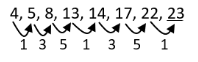Question 39
If the series 4,5,8,13,14,17,22,........ is continued in the same pattern, which one of the following is not a term of this series?
 A 31 B 32 C 33 D 35
Mathematical-Reasoning       Series-Test       2014 June UGC NET Paper 1
 Question 40
Complete the series BB, FE, II, ML, PP: ..:........by choosing one of the following option given :
 A TS B ST C RS D SR
Mathematical-Reasoning       Alphabet-Test       2014 June UGC NET Paper 1
Question 40 Explanation:
BB, FE, II, ML, PP, TS
After B we leave 3 and reach to F.
Similarly, after P leave 3 and reach T and one behind is S.
 Question 41
A man started walking frorn his house towards south. After walking 6 km, he turned to his left walked 5 Km after. Then he walked further 3 km after turning left. He then turned to his left and continued his walk for 9 km. How far is he away from his house?
 A 3 km B 4 km C 5 km D 6 km
Mathematical-Reasoning       Direction-Sense-Test       2014 June UGC NET Paper 1
Question 41 Explanation:
C2=42+32
C2=25
C=5Question 42
One writes all numbers from 50 to 99 without the digits 2 and 7. How many numbers have been written?
 A 32 B 36 C 40 D 38
Mathematical-Reasoning       Number-Theory       2014 June UGC NET Paper 1
 Question 43
The population of India is about 1.2 billion. Take the average consumption of energy per person per year in India as 30 Mega Joules. If this consumption is met by carbon based fuels and the rate of carbon emissions per kilojoule is l5 x I06 kgs, the total carbon emissions per year from India will be
 A 54 million metric tons B 540 million metric tons C 5400 million metric tons D 2400 million metric tons E None of These
Mathematical-Reasoning       Averages       2014 June UGC NET Paper 1
 Question 44
In a particular code, HOSPITALS is coded as HSOLSAPTI. The code of BIOLOGICALS will be
 A BLICOALIOSG B BOLGICAILOS C SBLAOILOBCG D BSILOALCOIG
Mathematical-Reasoning       Coding-and-Decoding       2016 August NTA UGC NET Paper 1
Question 44 Explanation:Question 45
In the series 1, 5, 13, 25, 41, ....... the next term is
 A 59 B 63 C 61 D 68
Mathematical-Reasoning       Series-Test       2016 August NTA UGC NET Paper 1
Question 45 Explanation: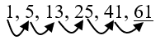Question 46
At present, a mother is 3 times older than her son. After 5 years, the sum of their ages will be 70 years. The age of the mother after 10 years will be
 A 40 B 55 C 45 D 60
Mathematical-Reasoning       Age-Related-Test       2016 August NTA UGC NET Paper 1
Question 46 Explanation:
M = 35
(M+5) + (S+5) = 70
M + S = 60
3S + S = 60
S = 15
M = 45
M + 10 = 55
 Question 47
In the series AYD, BVF, DRH, GMJ, ? the next term is
 A GLK B HLM C LHM D KGL
Mathematical-Reasoning       Alphabet-Test       2016 August NTA UGC NET Paper 1
Question 47 Explanation:Question 48
C and D are sisters. A and B are brothers. E is son of A and brother of D. B is related to C as
 A Brother B Son C Uncle D Father-in-law
Mathematical-Reasoning       Blood-Relation-Test       2016 August NTA UGC NET Paper 1
Question 48 Explanation:Question 49
Anil played 8 cricket matches. The mean (average) of the runs was found to be 80. After playing four more matches, the mean of the runs of all the matches was found to be 70. The total runs made in the last four matches is
 A 400 B 300 C 200 D 100
Mathematical-Reasoning       Averages       2016 August NTA UGC NET Paper 1
 Question 50
At present a person is 4 times older than his son and is 3 years older than his wife. After 3 years the age of the son will be 15 years. The age of the person's wife after 5 years will be:
 A 50 B 42 C 48 D 45
Mathematical-Reasoning       Age-Related-Test       2015 June NTA UGC Paper 1
Question 50 Explanation:
P = 45
P - 3 = W
S + 3 = 15
S = 12
P = 4 (12) = 48
W = P - 3 = 48 - 3 = 45
W + 5 = 45 + 5 = 50
 Question 51
In an examination 10,000 students appeared. The result revealed the number of students who have:
Passed in all five subjects = 5583
Passed in three subjects only = 1400
Passed in two subjects only = 1200
Passed in one subject only 735
Failed in English only = 75
Failed in Physics only = 145
Failed in Chemistry only = 140
Failed in Mathematics only = 200
Failed in Bio-science only = 157
The number of students passed in at least four subjects is:
 A 7900 B 6300 C 6900 D 7300
Mathematical-Reasoning       Averages       2015 June NTA UGC Paper 1
 Question 52
The next term in the series is:
2, 5, 9, 19, 37, ?
 A 80 B 73 C 75 D 78
Mathematical-Reasoning       Series-Test       2015 June NTA UGC Paper 1
Question 52 Explanation:
2 × 2+5=9
2 × 5+9=19
2 × 9+19=37
2 × 9+37=75
 Question 53
One day Prakash left home and walked 10 km towards south, turned right and walked 5 km, turned right and walked 10 km and turned left and walked 10 km. How many km will he have to walk to reach his home straight?
 A 30 B 10 C 20 D 15
Mathematical-Reasoning       Direction-Sense-Test       2015 June NTA UGC Paper 1
Question 53 Explanation:Question 54
A girl introduced a boy as the son of the daughter of the father of her uncle. The boy is related to the girl as:
 A Son B Brother C Uncle D Nephew
Mathematical-Reasoning       Blood-Relation-Test       2015 June NTA UGC Paper 1
 Question 55
In certain code MATHURA is coded as JXQEROX. The code of HOTELS will be:
 A ELIPQB B LEQIBP C ELQBIP D LEBIQP
Mathematical-Reasoning       Coding-and-Decoding       2015 June NTA UGC Paper 1
Question 55 Explanation:Question 56
A person walks 10 m in front and 10 m to the right. Then every time turning to his left, he walks 5, 15 and 15 m respectively. How far is he from his starting point?
 A 20 m B 15 m C 10 m D 5 m
Mathematical-Reasoning       Direction-Sense-Test       2016 July NTA UGC NET Paper 1
 Question 57
A sister of B. F is daughter of G. C is mother of B. D is father of C. E is mother of D. A is related to D as
 A Grand daughter B Daughter C Daughter-in-law D Sister
Mathematical-Reasoning       Blood-Relation-Test       2016 July NTA UGC NET Paper 1
 Question 58
In the series AB, EDC, FGHI, ________? ________ OPQRST, The missing term is
 A JKLMN B JMKNL C NMLKJ D NMKLJ
Mathematical-Reasoning       Alphabet-Test       2016 July NTA UGC NET Paper 1
 Question 59
In certain code, SELECTION is coded as QCJCARGML. The code of AMERICANS will be
 A YKCPGAYLQ B BNFSJDBMR C QLYAGPCKY D YQKLCYPAG
Mathematical-Reasoning       Coding-and-Decoding       2016 July NTA UGC NET Paper 1
 Question 60
In the series 3, 11, 23, 39, 59, ________ The next term will be
 A 63 B 73 C 83 D 93
Mathematical-Reasoning       Series-Test       2016 July NTA UGC NET Paper 1
 Question 61
Two railway tickets from city A and B three ticketd from city A to C cost Rs. 177. Three tickets from city A to B and two tickets from city A to C city Rs. 173. The fare for city B from city A will be Rs.
 A 25 B 27 C 30 D 33
Mathematical-Reasoning       Distance-and-Time       2016 July NTA UGC NET Paper 1
Question 61 Explanation:
Let P=Ticket from A to B
Q=Ticket from A to C
According to given data,
2P + 3Q = 177 --> 1
3P + 2Q= 173 --> 2

Multiply 1 by 3 and 2 by 2
6P + 9Q = 531
6P + 4Q = 346
- - -
-----------------------
5Q = 185
Q= 37
Substitute value of Q in 1
P=33
 Question 62
The next term in the series 2, 5, 10, 17, 26, 37, ? is:
 A 50 B 57 C 62 D 72
Mathematical-Reasoning       Series-Test       2015 December NTA UGC NET Paper 1
Question 62 Explanation: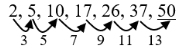Question 63
A group of 210 students appeared in some test. The mean of 1/3 rd of students is found to be 60. The mean of the remaining students is found to be 78. The mean of the whole group will be:
 A 80 B 76 C 74 D 72
Mathematical-Reasoning       Averages       2015 December NTA UGC NET Paper 1
Question 63 Explanation:
Given data:
Total number of students = 210
1/3 rd of 210 = 70 students
Mean marks of 70 students = 60
To Find: Mean of the whole group= ?
Let the sum of marks of 70 students be X
Mean = X / Number of students
60 = X/70
X = 70*60

⇒ Sum of marks of 70 students = 4200
Remaining students = 210 - 70 = 140 students
Mean of 140 students = 78
Sum of marks of 78 students = 140*78 = 10920
Total marks of 210 students = 4200 + 10920
= 15120
Mean of whole group = 15120 / 210
= 72 marks
 Question 64
Anil after travelling 6 km towards East from his house realized that he has travelled in a wrong direction, lie turned and travelled 12 km towards West, turned right and travelled 8 km to reach his office. The straight distance of the office from his house is:
 A 20 km B 14 km C 12 km D 10 km
Mathematical-Reasoning       Direction-Sense-Test       2015 December NTA UGC NET Paper 1
Question 64 Explanation:Question 65
The next term in the series: B2E, D5H, F12K, H27N, ? is:
 A J561 B 162Q C Q62J D J58Q
Mathematical-Reasoning       Alphabet-Test       2015 December NTA UGC NET Paper 1
Question 65 Explanation:Question 66
The next term in the following series YEB, WFD, UHG, SKI, ? will be
 A TLO B QOL C QLO D GQP
Mathematical-Reasoning       Alphabet-Test       2017 January NTA UGC NET Paper 1
Question 66 Explanation:Question 67
If A is coded as C, M as I, N as P, S as 0, I as A, P as N, E as M, 0 as E and C as S, then the code of COMPANIES will be
 A SPEINMOAC B NCPSEIOMA C SMOPIEACN D SEINCPAMO
Mathematical-Reasoning       Coding-and-Decoding       2017 January NTA UGC NET Paper 1
Question 67 Explanation: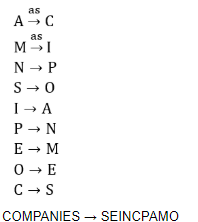Question 68
Ali buys a glass, a pencil box and a cup and pays Rs. 21 to the shopkeeper. Rakesh buys a cup, two pencil boxes and a glass and pays Rs. 28 to the shopkeeper. Preeti buys two glasses, a cup and two pencil boxes and pays Rs. 35 to the shopkeeper. The cost of 10 cups will be
 A Rs.40 B Rs.60 C Rs.80 D Rs.70
Mathematical-Reasoning       Profit-and-Loss       2017 January NTA UGC NET Paper 1
Question 68 Explanation:Question 69
Out of four cities given below three are alike in some manner while the fourth one is different. Identify the odd one
 A Lucknow B Rishikesh C Allahabad D Patna
Mathematical-Reasoning       Classification       2017 January NTA UGC NET Paper 1
Question 69 Explanation:
River ganga doesn’t pass through LUCKNOW.
 Question 70
The missing term in the series 1, 4, 27, 16, ?, 36, 343, ... is
 A 30 B 49 C 125 D 81
Mathematical-Reasoning       Series-Test       2017 January NTA UGC NET Paper 1
Question 70 Explanation: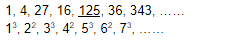Question 71
The next term in the series 5, 11, 21, 35, 53, ?, ... is
 A 75 B 90 C 115 D 125
Mathematical-Reasoning       Series-Test       2018 July 22 NTA UGC NET Paper 1
 Question 72
The next term in the series XY, ABC, FGHI, ? , ... is
 A MNPQO B MNOPQ C PQOMN D NMPOQ
Mathematical-Reasoning       Alphabet-Test       2018 July 22 NTA UGC NET Paper 1
 Question 73
If the code of ALLAHABAD is DPQGOIKKO, then the code of BENGULURU will be
 A ESBTBDIMF B MBDBFEIST C EISMBTDBF D ESBDFBTMI
Mathematical-Reasoning       Coding-and-Decoding       2018 July 22 NTA UGC NET Paper 1
 Question 74
A man paid Rs. 160 while travelling 10 km in a taxi which has some initial fixed charges. Another man paid Rs. 276 for travelling 16 km and the taxi driver charged double of the initial fixed charges from him. The charges of the taxi per km is
 A Rs. 10 B Rs. 13 C Rs. 11 D Rs. 17
Mathematical-Reasoning       Distance-and-Time       2018 July 22 NTA UGC NET Paper 1
 Question 75
Gopal walks 20 m North. Then he turns right and walks 30 m. Then he turns right and walks 35 m. Again he turns left and walks 15 m. Then he again turns left and walks 15 m. The shortest distance between his original position and final one is
 A 65 m B 55 m C 40 m D 45 m
Mathematical-Reasoning       Direction-Sense-Test       2018 July 22 NTA UGC NET Paper 1
 Question 76
Choose the proper alternative given in the code to replace the question mark.
Cow – Milk
Bee – Honey
Teacher – ?
 A Marks B Discipline C Wisdom D Lesson
Mathematical-Reasoning       Analogy-Test       2018 July 22 NTA UGC NET Paper 1
 Question 77
The next term in the series ABD, DGK, HMS, MTB ... is:
 A SBL B PSK C RUH D NSA
Mathematical-Reasoning       Alphabet-Test       2017 November NTA UGC NET Paper 1
Question 77 Explanation:Question 78
In certain code, “COVALENT” is code as BWPDUOFM. The code of “ELEPHANT” will be:
 A QMUBIADH B QFMFUOBI C EPHNTEAS D MFUIQRTW
Mathematical-Reasoning       Coding-and-Decoding       2017 November NTA UGC NET Paper 1
Question 78 Explanation:Question 79
In the series 1, 6, 15, 28, 45 ... the next term will be:
 A 76 B 56 C 84 D 66
Mathematical-Reasoning       Series-Test       2017 November NTA UGC NET Paper 1
Question 79 Explanation:Question 80
Ajay is a friend of Rakesh, Pointing to an old man Ajay asked Rakesh who is he? Rakesh said “His son is my son’s uncle”. The old man is related to Rakesh as:
 A Father-in law B Father C Uncle D Grandfather
Mathematical-Reasoning       Blood-Relation-Test       2017 November NTA UGC NET Paper 1
 Question 81
A postman walked 20 m straight from his office, turned right and walked 10 m. After turning left he walked 10 m and after turning right walked 20 m. He again turned right and walked 70m. How far he is from his office?
 A 40 m B 60 m C 20 m D 50 m
Mathematical-Reasoning       Direction-Sense-Test       2017 November NTA UGC NET Paper 1
 Question 82
Insert the missing number :
8 24 12 ? 18 54
 A 26 B 24 C 36 D 32
Mathematical-Reasoning       Series-Test       2005 Dec UGC NET Paper-1
Question 82 Explanation:Question 83
January 1, 1995 was Sunday. What day of the week lies on January 1, 1996 ?
 A Sunday B Monday C Saturday D None of these
Mathematical-Reasoning       Calendar       2005 Dec UGC NET Paper-1
Question 83 Explanation:
No. of odd days between Jan 1st 1995 to Jan 1st 1996 is 1.
∴ Jan 1st, 1996 is Monday.
 Question 84
The sum of a positive number and its reciprocal is twice the difference of the number and its reciprocal. The number is :
 A √2 B 1/ √2 C √3 D 1/ √3
Mathematical-Reasoning       Number-Theory       2005 Dec UGC NET Paper-1
Question 84 Explanation:Question 85
In a certain code, ROUNDS is written as RONUDS. How will PLEASE will be written in the same code :
 A L PA E S E B P L A E S E C L P A E E S D P L A S E E
Mathematical-Reasoning       Coding-and-Decoding       2005 Dec UGC NET Paper-1
Question 85 Explanation:Question 86
At what time between 5.30 and 6.00 will the hands of an clock be at right angles ?
 A 43 (5/11) min. past 5 B 43 (7/11) min. past 5 C 40 min. past 5 D 45 min past 5
Mathematical-Reasoning       Clock-Related-Test       2005 Dec UGC NET Paper-1
Question 86 Explanation: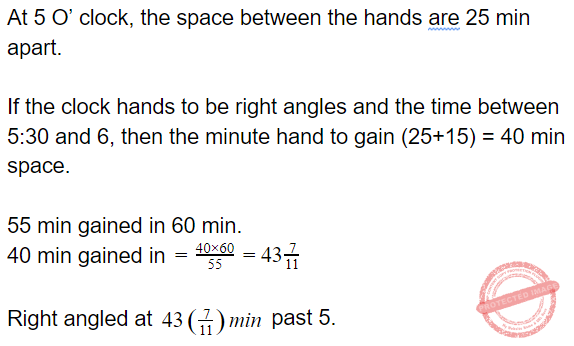Question 87
What will be the next letter in the following series? DCXW, FGVU, HGTS
 A AKPO B JBYZ C JIRQ D LMRS
Mathematical-Reasoning       Alphabet-Test       2008 June UGC NET Paper 1
Question 87 Explanation:Question 88
AGARTALA is written in code as 14168171, the code for AGRA is:
 A 1641 B 1416 C 1441 D 1461
Mathematical-Reasoning       Coding-and-Decoding       2008 June UGC NET Paper 1
Question 88 Explanation:Question 89
If January 1st, 2007 is Monday, what was the day on 1st January 1995?
 A Sunday B Monday C Friday D Saturday
Mathematical-Reasoning       Calendar       2008 June UGC NET Paper 1
Question 89 Explanation:
Initially calculate all odd days in each year.
1995 → 1 odd day
1996 → 2 odd day (leap year)
1997, 1998, 1999 → (1 + 1 + 1) odd days
2000 → 2 odd days
2001+2002+2003 → 3 odd days
2004 → 2 odd days
2005+2006 → (1 + 1) odd days
Total odd days = 15 odd days = 1 odd day
Such that : 1st January 1995 = Sunday
 Question 90
Insert the missing number in the following series: 4          16        8          64        ?          256
 A 16 B 24 C 32 D 20
Mathematical-Reasoning       Series-Test       2008 June UGC NET Paper 1
Question 90 Explanation:Question 91
If an article is sold for Rs. 178 at a loss of 11%; what would be its selling price in order to earn a profit of 11%?
 A Rs. 222.50 B Rs. 267 C Rs. 222 D Rs. 220
Mathematical-Reasoning       Profit-and-Loss       2008 June UGC NET Paper 1
Question 91 Explanation: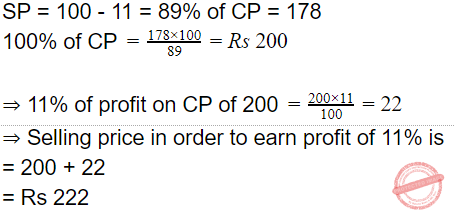Question 92
Which of the following statements is not connected with communication?
 A Medium is the message. B The world is an electronic cocoon. C Information is power. D Telepathy is technological.
Mathematical-Reasoning       Series-Test       2009 December UGC NET Paper 1
 Question 93
January 1, 1995 was a Sunday. What day of the week lies on January 1, 1996?
 A Sunday B Monday C Wednesday D Saturday
Mathematical-Reasoning       Communication-Assertion-Reason       2009 December UGC NET Paper 1
Question 93 Explanation:
1995 is an ordinary year. So it has only one odd day. So 1st january 1996 will be one day beyond Sunday i.e., Monday.
 Question 94
When an error of 1% is made in the length and breadth of a rectangle, the percentage error (%) in the area of a rectangle will be
 A 0 B 1 C 2 D 4
Mathematical-Reasoning       Percentage       2009 December UGC NET Paper 1
 Question 95
The next number in the series 2, 5, 9, 19, 37, ? will be
 A 74 B 75 C 76 D None of the above
Mathematical-Reasoning       Series-Test       2009 December UGC NET Paper 1
Question 95 Explanation:Question 96
There are 10 true-false questions in an examination. Then these questions can be answered in
 A 20 ways B 100 ways C 240 ways D 1024 ways
Mathematical-Reasoning       Permutation-and-Combination       2009 December UGC NET Paper 1
Question 96 Explanation:
No. of questions = 10
No. of options = 2
No. of possible ways = 210 = 1024
 Question 97
What will be the next term in the following? DCXW, FEVU, HGTS, ?
 A AKPO B ABYZ C JIRQ D LMRS
Mathematical-Reasoning       Alphabet-Test       2009 December UGC NET Paper 1
Question 97 Explanation:Question 98
Three individuals X, Y, Z hired a car on a sharing basis and paid Rs. 1,040. They used it for 7, 8, 11 hours, respectively. What are the charges paid by Y?
 A Rs. 290 B Rs. 320 C Rs. 360 D Rs. 440
Mathematical-Reasoning       Partnership       2009 December UGC NET Paper 1
Question 98 Explanation: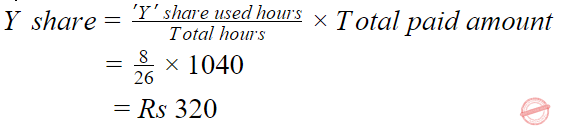Question 99
In the sequence of numbers 8, 24, 12, X, 18, 54 the missing number X is:
 A 26 B 24 C 36 D 32
Mathematical-Reasoning       Series-Test       2006 December UGC NET Paper 1
Question 99 Explanation: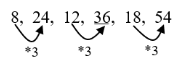Question 100
If A stands for 5, B for 6, C for 7, D for 8 and so on, then the following numbers stand for 17, 19, 20, 9 and 8:
 A PLANE B MOPED C MOTOR D TONGA
Mathematical-Reasoning       Coding-and-Decoding       2006 December UGC NET Paper 1
Question 100 Explanation:
A = 5, B = 6, C = 7, D = 8, …
A = (1+4), B = (2+4), C = (3 + 4), D = (4+4) …….
17, 19, 20, 9, 8 = (13+4), (15+4), (16+4), (5+4), (4+4)
= MOPED
 Question 101
The letters in the first set have certain relationship. On the basis of this relationship, what is the right choice for the second set? AST : BRU : : NQV : ?
 A ORW B MPU C MRW D OPW
Mathematical-Reasoning       Analogy-Test       2006 December UGC NET Paper 1
Question 101 Explanation:Question 102
In a certain code, PAN is written as 31 and PAR as 35. In this code PAT is written as:
 A 30 B 37 C 38 D 39
Mathematical-Reasoning       Coding-and-Decoding       2006 December UGC NET Paper 1
Question 102 Explanation:Question 103
The sides of a triangle are in the ratio of   1/2 : 1/3 : 1/4.  If its perimeter is 52 cm, the length of the smallest side is:
 A 9 cm B 10 cm C 11 cm D 12 cm
Mathematical-Reasoning       Geometry       2006 December UGC NET Paper 1
Question 103 Explanation: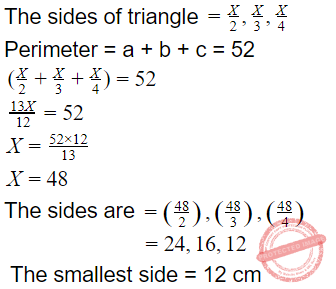Question 104
Which of the following statements say the same thing?
(i) “I am a teacher” (said by Arvind)
(ii) “I am a teacher” (said by Binod)
(iii) “My son is a teacher” (said by Binod’s father)
(iv) “My brother is a teacher” (said by Binod’s sister)
(v) “My brother is a teacher” (said by Binod’s only sister)
(vi) “My sole enemy is a teacher” (said by Binod’s only enemy)
Choose the correct answer from the code given below:
 A (i) and (ii) B (ii), (iii), (iv) and (v) C (ii) and (vi) D (v) and (vi)
Mathematical-Reasoning       Blood-Relation-Test       2006 December UGC NET Paper 1
Question 104 Explanation:
(ii), (iii), (iv), (v) and (vi) statements are saying that Binod is a teacher from the options ‘B’ is correct.
 Question 105
Which of the following are correct ways of arguing?
(i) There can be no second husband without a second wife.
(ii) Anil is a friend of Bob, Bob is a friend of Raj, hence Anil is a friend of Raj.
(iii) A is equal to B, B is equal to C, hence A is equal to C.
(iv) If everyone is a liar, then we cannot prove it.
Choose the correct answer from the code given below:
 A (iii) and (iv) B (i), (iii) and (iv) C (ii), (iii) and (iv) D (i), (ii), (iii) and (iv)
Mathematical-Reasoning       Blood-Relation-Test       2006 December UGC NET Paper 1
Question 105 Explanation:
Argument:- An exchange of diverging (or) opposite views. Statements (iii) and (iv) are arguments to each other.
 Question 106
Insert the missing number: 16/32,             15 /33,             17/31,              14/34               ?
 A 19/35 B 19/30 C 18/35 D 18/30
Mathematical-Reasoning       Series-Test       2006 June UGC NET Paper-1
Question 106 Explanation:Question 107
Monday falls on 20th March 1995.  What was the day on 3rd November 1994?
 A Thursday B Sunday C Tuesday D Saturday
Mathematical-Reasoning       Calendar       2006 June UGC NET Paper-1
Question 107 Explanation:
Initially calculate odd days.
3rd November - 30 November = 27 days
In December = 31
In January = 31
In February = 28
March 1st - 20th March = 20
Total days = 137
= 4 odd days
The 3rd November 1994 = Thursday
 Question 108
The average of four consecutive even numbers is 27. The largest of these numbers is:
 A 36 B 32 C 30 D 28
Mathematical-Reasoning       Averages       2006 June UGC NET Paper-1
Question 108 Explanation:Question 109
In a certain code, FHQK means GIRL. How will WOMEN be written in the same code?
 A VNLDM B FHQKN C XPNFO D VLNDM
Mathematical-Reasoning       Coding-and-Decoding       2006 June UGC NET Paper-1
Question 109 Explanation:Question 110
At what time between 4 and 5 O'clock will the hands of a watch point in opposite directions?
 A 45 min. past 4 B 40 min. past 4 C 50 4/11 min. past 4 D 54 6/11 min. past 4
Mathematical-Reasoning       Clock-Related-Test       2006 June UGC NET Paper-1
Question 110 Explanation:Question 111
Which number is missing in the following series? 2, 5, 10, 17, 26, 37, 50, ?
 A 63 B 65 C 67 D 69
Mathematical-Reasoning       Series-Test       2007 December UGC NET Paper 1
 Question 112
Insert the missing number:   4 : 17 : : 7 : ?
 A 48 B 49 C 50 D 51
Mathematical-Reasoning       Analogy-Test       2007 December UGC NET Paper 1
Question 112 Explanation:Question 113
Choose the odd word:
 A Nun B Knight C Monk D Priest
Mathematical-Reasoning       Classification-Test       2007 December UGC NET Paper 1
 Question 114
Choose the number which is different from others in the group:
 A 49 B 63 C 77 D 81
Mathematical-Reasoning       Classification-Test       2007 December UGC NET Paper 1
Question 114 Explanation:Question 115
Insert the missing number:      ______36/62 ,  39/63,  43/61, 48/64, ?
 A 51/65 B 56/60 C 54/65 D 33/60
Mathematical-Reasoning       Series-Test       2007 December UGC NET Paper 1
Question 115 Explanation: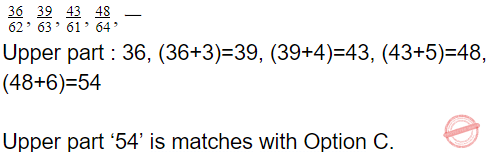Question 116
At what time between 3 and 4 O'clock will the hands of a watch point in opposite directions?
 A 40 minutes past three B 50 minutes past three C 45 minutes past three D 55 minutes past three
Mathematical-Reasoning       Clock-Related-Test       2007 December UGC NET Paper 1
 Question 117
Mary has three children. What is the probability that none of the three children is a boy?
 A 1/2 B 1/3 C 3/4 D 1 E None of The Above
Mathematical-Reasoning       Probability       2007 December UGC NET Paper 1
Question 117 Explanation:
(Wrong question)
Description:
1. The probably of a boy are bbb, bbg, bgb, gbb, bgg, gbg, ggb, ggg, which is 8 'equally likely' cases, one meets desired criteria, probability of desired criteria is 1/8 .
2. Probability that first child is not boy= 1/2
Probability that second child is not boy= 1/2
Probability that third child is not boy= 1/2
Probability that none of the three children is a boy= 1/2 x 1/2 x 1/2= 1/8
 Question 118
If the radius of a circle is increased by 50 per cent. Its area is increased by:
 A 125 per cent B 100 per cent C 75 per cent D 50 per cent
Mathematical-Reasoning       Geometry       2007 December UGC NET Paper 1
 Question 119
Which is the number that comes next in the sequence? 9, 8, 8, 8, 7, 8, 6,
 A 5 B 6 C 8 D 4
Mathematical-Reasoning       Series-Test       2007 June UGC NET Paper 1
Question 119 Explanation:Question 120
If in a certain language TRIVANDRUM is coded as 2 5 9 5 3 5 4 7 5 8, how would MADRAS be coded?
 A 834536 B 834538 C 834530 D 834539
Mathematical-Reasoning       Coding-and-Decoding       2007 June UGC NET Paper 1
 Question 121
January 12, 1980 was Saturday, what day was January 12, 1979:
 A Saturday B Friday C Sunday D Thursday
Mathematical-Reasoning       Calendar       2007 June UGC NET Paper 1
Question 121 Explanation:
No. of odd days from Jan 12, 1979 to Jan 12, 1980 is = “i” So January 12, 1979 is friday.
 Question 122
How many Mondays are there in a particular month of a particular year, if the month ends on Wednesday?
 A 5 B 4 C 3 D None of the above
Mathematical-Reasoning       Calendar       2007 June UGC NET Paper 1
Question 122 Explanation:
If month ends with wednesday then possible dates of month end is 31, 30, 28.
The possible dates of monday = 29, 28, 26
If last monday 29 then possible dates on monday = 29, 22, 15, 8, 1
If 28th then = 28, 21, 14, 7
If 26th then = 26, 19, 12, 5

Min possible mondays = 4
 Question 123
'A' is the father of 'C, and 'D' is the son of 'B'. 'E' is the brother of 'A'. If 'C is the sister of 'D', how is 'B' related to 'E'?
 A daughter B husband C sister-in-law D brother-in-law
Mathematical-Reasoning       Blood-Relation-Test       2007 June UGC NET Paper 1
Question 123 Explanation:
A is father of C and C is sister of D. So A is father of D.
But D is son of B. So, B is mother of D and wife of K also.
E is the brother of A.
So B is the sister-in-law of E.
 Question 124
A ratio represents the relation between:
 A Part and Part B Part and Whole C Whole and Whole D All of the above
Mathematical-Reasoning       Ratio-and-Proportion       2007 June UGC NET Paper 1
 Question 125
Out of four numbers, the average of the first three numbers is thrice the fourth number. If the average of the four numbers is 5, the fourth number is:
 A 4.5 B 5 C 2 D 4
Mathematical-Reasoning       Averages       2007 June UGC NET Paper 1
 Question 126
Insert the missing number in the following: 2/3, 4/7, ? , 11/21, 16/31
 A 10/8 B 6/10 C 5/10 D 7/13
Mathematical-Reasoning       Series-Test       2008 December UGC NET Paper 1
Question 126 Explanation:Question 127
In a certain code, GAMESMAN is written as AGMEMSAN.   How would DISCLOSE be written in that code?
 A IDSCOLSE B IDCSOLES C IDSCOLES D IDSCLOSE
Mathematical-Reasoning       Coding-and-Decoding       2008 December UGC NET Paper 1
Question 127 Explanation: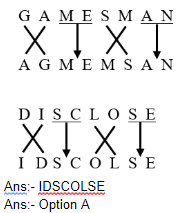Question 128
The letters in the first set have a certain relationship.  On the basis of this relationship mark the right choice for the second set: AST : BRU : : NQV : ?
 A ORW B MPU C MRW D OPW
Mathematical-Reasoning       Alphabet-Test       2008 December UGC NET Paper 1
Question 128 Explanation:Question 129
On what dates of April 1994 did SUNDAY fall?
 A 2,9,16,23,30 B 3,10,17,24 C 4,11,18,25 D 1,8,15,22,29
Mathematical-Reasoning       Calendar       2008 December UGC NET Paper 1
Question 129 Explanation:
1600 Years contains ‘0’ odd day.
300 Years contains ‘1’ odd day.
93 Years = 23 leap years + 70 ordinary years
= (46 + 70) odd days
= 116 odd days
= 4 odd days

No. of days upto 1st April, 1994
31 + 28 + 31 + 1 = 91 days [0 odd days]
Total odd days = (0 + 1 + 4 + 0) = 5
Day on 1st April is (Sunday + 5) = Friday
The fridays fall on = 1, 8, 15, 22, 29
The Sundays fall on = (1+2), (8+2), (15+2), (22+2), (29+2)
=3,10,17,24,31 (April have only sundays)
 Question 130
Find out the wrong number in the sequence:
125, 127, 130, 135, 142, 153, 165
 A 130 B 142 C 153 D 165
Mathematical-Reasoning       Series-Test       2008 December UGC NET Paper 1
Question 130 Explanation:Question 131
There are five books A, B, C, D and E.  The book C lies above D, the book E is below A and B is below E. Which is at the bottom?
 A E B B C A D C
Mathematical-Reasoning       Seating-Arrangement       2008 December UGC NET Paper 1
 Question 132
In a certain code, CLOCK is written as KCOLC. How would STEPS be written in that code?
 A SPEST B SPSET C SPETS D SEPTS
Mathematical-Reasoning       Coding-and-Decoding       2009 June UGC NET Paper 1
Question 132 Explanation:
Clock is written as KCOLK which is reverse order same as reverse order of STEPS will be SPETS.
 Question 133
The letters in the first set have a certain relationship. On the basis of this relationship mark the right choice for the second set: BDFH : OMKI : : GHIK : ?
 A FHJL B RPNL C LNPR D LJHF
Mathematical-Reasoning       Alphabet-Test       2009 June UGC NET Paper 1
Question 133 Explanation:Question 134
What was the day of the week on 1st January 2001?
 A Friday B Tuesday C Sunday D Wednesday E None of the Above
Mathematical-Reasoning       Calendar       2009 June UGC NET Paper 1
Question 134 Explanation:
(Wrong question)
 Question 135
Find out the wrong number in the sequence. 52, 51, 48, 43, 34, 27, 16
 A 27 B 34 C 43 D 48
Mathematical-Reasoning       Series-Test       2009 June UGC NET Paper 1
Question 135 Explanation:‘34’ is the wrong number the order to be like increasing of 1, 3, 5, 7, 9, 11 instead of 34, the correct number is 36.
 Question 136
Two ladies and two men are playing bridge and seated at North, East, South and West of a table. No lady is facing East. Persons sitting opposite to each other are not of the same sex.  One man is facing South.  Which direction are the ladies facing to?
 A East and West B North and West C South and East D None of these
Mathematical-Reasoning       Seating-Arrangement       2009 June UGC NET Paper 1
 Question 137
Determine the relationship between the pair of words ALWAYS : NEVER and then select from the following pair of words which have a similar relationship :
 A often : rarely B frequently : occasionally C constantly : frequently D intermittently : casually
Mathematical-Reasoning       Analogy-Test       2010 December UGC NET Paper 1
 Question 138
Find the wrong number in the sequence: 52, 51, 48, 43, 34, 27, 16
 A 27 B 34 C 43 D 48
Mathematical-Reasoning       Series-Test       2010 December UGC NET Paper 1
Question 138 Explanation: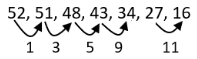‘34’ is wrong number in place of 34, the number ‘36’ is correct sequence.
 Question 139
In a certain code, PAN is written as 31 and PAR as 35, then PAT is written in the same code as
 A 30 B 37 C 39 D 41
Mathematical-Reasoning       Coding-and-Decoding       2010 December UGC NET Paper 1
Question 139 Explanation:
PAN = 31 → (1)
PAR = 35 → (2)
PAT = 37
From (1) & (2), PA = 3; Difference between N to R is (5 - 1) = 4 i.e., no. of alphabets between N & R such as no. of alphabets between R and T is ‘2’ then ‘7’ is correct number. Difference between 7 and 5 is 2.
 Question 140
The letters in the first set have certain relationship. On the basis of this relationship, make the right choice for the second set: AF : IK : : LQ : ?
 A MO B NP C OR D TV
Mathematical-Reasoning       Alphabet-Test       2010 December UGC NET Paper 1
Question 140 Explanation:Question 141
If 5472 = 9, 6342 = 6, 7584 = 6, what is 9236?
 A 2 B 3 C 4 D 5
Mathematical-Reasoning       Coding-and-Decoding       2010 December UGC NET Paper 1
Question 141 Explanation:
5 + 4 + 7 + 2 = 18 = (1 + 8) = 9; 6 + 3 + 4 + 2 = 15 = (1 + 5) = 6
7 + 5 + 8 + 4 = 24 = (2 + 4) = 6; 9 + 2 + 3 + 6 = 20 = (2 + 0) = 2
 Question 142
What is the smallest number of ducks that could swim in this formation – two ducks in front of a duck, two ducks behind a duck and a duck between two ducks?
 A 5 B 7 C 4 D 3
Mathematical-Reasoning       Simple-Aptitude       2010 December UGC NET Paper 1
 Question 143
Mr. A, Miss B, Mr. C and Miss D are sitting around a table and discussing their trades. (i) Mr. A sits opposite to the cook. (ii) Miss B sits right to the barber (iii) The washerman sits right to the barber (iv) Miss D sits opposite to Mr. C What are the trades of A and B?
 A Tailor and barber B Barber and cook C Tailor and cook D Tailor and washerman
Mathematical-Reasoning       Seating-Arrangement       2010 December UGC NET Paper 1
 Question 144
Which one of the following methods serve to measure correlation between two variables?
 A Scatter Diagram B Frequency Distribution C Two-way table D Coefficient of Rank Correlation
Mathematical-Reasoning       Geometry       2010 December UGC NET Paper 1
 Question 145
When an error of 1% is made in the length of a square, the percentage error in the area of a square will be
 A 0 B 1/2 C 1 D 2
Mathematical-Reasoning       Percentage       2010 June UGC NET Paper 1
 Question 146
On January 12, 1980, it was a Saturday.  The day of the week on January 12, 1979 was
 A Thursday B Friday C Saturday D Sunday
Mathematical-Reasoning       Calendar       2010 June UGC NET Paper 1
 Question 147
If water is called food, food is called tree, tree is called earth, earth is called world, which of the following grows a fruit?
 A Water B Tree C World D Earth
Mathematical-Reasoning       Coding-and-Decoding       2010 June UGC NET Paper 1
Question 147 Explanation:
Water = food
Food = tree
Tree = earth
Earth = world
Fruits glows in a tree. Tree is called as earth.
Ans:- C
 Question 148
E is the son of A, D is the son of B, E is married to C, C is the daughter of B. How is D related to E?
 A Brother B Uncle C Father-in-law D Brother-in-law
Mathematical-Reasoning       Blood-Relation-Test       2010 June UGC NET Paper 1
Question 148 Explanation:
C is D’s daughter and D is B’s son.
So, D is the brother of C. E is a male married to C. So, E is husband of C, whose brother is D.
So, D is the brother-in-law of E.
 Question 149
If INSURANCE is coded as ECNARUSNI, how HINDRANCE will be coded?
 A CADNIHWCE B HANODEINR C AENIRHDCN D ECNARDNIH
Mathematical-Reasoning       Coding-and-Decoding       2010 June UGC NET Paper 1
Question 149 Explanation:
INSURANCE write inverse order ECNARUSNI such as HINDRANCE can coded as ECNARDNIH.
 Question 150
Find the next number in the following series: 2, 5, 10, 17, 26, 37, 50, ?
 A 63 B 65 C 67 D 69
Mathematical-Reasoning       Series-Test       2010 June UGC NET Paper 1
Question 150 Explanation:Question 151
Which of the following is an example of circular argument?
 A God created man in his image and man created God in his own image. B God is the source of a scripture and the scripture is the source of our knowledge of God. C Some of the Indians are great because India is great. D Rama is great because he is Rama.
Mathematical-Reasoning       Seating-Arrangement       2010 June UGC NET Paper 1
 Question 152
Lakshmana is a morally good person because
 A he is religious B he is educated C he is rich D he is rational
Mathematical-Reasoning       Analogy-Test       2010 June UGC NET Paper 1
 Question 153
The population of a city is plotted as a function of time (years) in graphic form below:Which of the following inference can be drawn from above plot?
 A The population increases exponentially. B The population increases in parabolic fashion. C The population initially increases in a linear fashion and then stabilizes. D The population initially increases exponentially and then stabilizes.
Mathematical-Reasoning       Geometry       2010 June UGC NET Paper 1
 Question 154
What is the number that comes next in the sequence? 2, 5, 9, 19, 37, ___
 A 76 B 74 C 75 D 50
Mathematical-Reasoning       Series-Test       2011 December UGC NET Paper 1
Question 154 Explanation:
2, 5, 9, 19, 37, 75
22+1=5
52-1=9
92+1=19
192-1=37
372+1=75
 Question 155
Find the next letter for the series MPSV..…
 A X B Y C Z D A
Mathematical-Reasoning       Alphabet-Test       2011 December UGC NET Paper 1
Question 155 Explanation:Question 156
If ‘367’ means ‘I am happy’; ‘748’ means ‘you are sad’ and ‘469’ means ‘happy and sad’ in a given code, then which of the following represents ‘and’ in that code ?
 A 3 B 6 C 9 D 4
Mathematical-Reasoning       Coding-and-Decoding       2011 December UGC NET Paper 1
Question 156 Explanation:
367 = I am happy
6 = Happy, 4 = sad; then 6 = and
 Question 157
The basis of the following classification is ‘animal’, ‘man’, ‘house’, ‘book’, and ‘student’:
 A Definite descriptions B Proper names C Descriptive phrases D Common names
Mathematical-Reasoning       Classification       2011 December UGC NET Paper 1
 Question 158
If the side of the square increases by 40%, then the area of the square increases by
 A 60 % B 40 % C 196 % D 96 %
Mathematical-Reasoning       Geometry       2011 December UGC NET Paper 1
Question 158 Explanation:
Area of square =a2 a is length of side The area of a side increased by 40%.
Let assume side = 100 then Area = 10,000
Side increased by 40% = 140
Then Area = 140 × 140 = 19600
Area of square increase =19600-1000010000100=96%
 Question 159
There are 10 lamps in a hall. Each one of them can be switched on independently. The number of ways in which hall can be illuminated is
 A 102 B 1023 C 210 D 10 !
Mathematical-Reasoning       Permutation-and-Combination       2011 December UGC NET Paper 1
 Question 160
How many numbers between 100 and 300 begin or end with 2?
 A 100 B 110 C 120 D 180
Mathematical-Reasoning       Number-Theory       2011 December UGC NET Paper 1
 Question 161
In a college having 300 students, every student reads 5 newspapers and every newspaper is read by 60 students. The number of newspapers required is
 A at least 30 B at most 20 C exactly 25 D exactly 5
Mathematical-Reasoning       Number-Theory       2011 December UGC NET Paper 1
 Question 162
Determine the relationship between the pair of words NUMERATOR : DENOMINATOR and then select the pair of words from the following which have a similar relationship :
 A fraction : decimal B divisor : quotient C top : bottom D dividend : divisor
Mathematical-Reasoning       Analogy-Test       2011 June UGC NET Paper 1
 Question 163
Find the wrong number in the sequence 125, 127, 130, 135, 142, 153, 165
 A 130 B 142 C 153 D 165
Mathematical-Reasoning       Series-Test       2011 June UGC NET Paper 1
 Question 164
The letters in the first set have certain relationship. On the basis of this relationship, make the right choice for the second set: K/T : 11/20 :: J/R : ?
 A 10/8 B 10/18 C 11/19 D 10/19
Mathematical-Reasoning       Alphabet-Test       2011 June UGC NET Paper 1
 Question 165
If A = 5, B = 6, C = 7, D = 8 and so on, what do the following numbers stand for? 17, 19, 20, 9, 8
 A Plane B Moped C Motor D Tonga
Mathematical-Reasoning       Alphabet-Test       2011 June UGC NET Paper 1
 Question 166
The price of oil is increased by 25%.  If the expenditure is not allowed to increase, the ratio between the reduction in consumption and the original consumption is
 A 1 : 3 B 1 : 4 C 1 : 5 D 1 : 6
Mathematical-Reasoning       Percentage       2011 June UGC NET Paper 1
 Question 167
How many 8’s are there in the following sequence which are preceded by 5 but not immediately followed by 3 ? 5  8  3  7  5  8  6  3  8  5  4  5  8  4  7  6 5  5  8  3  5  8  7  5  8  2  8  5
 A 4 B 5 C 7 D 3
Mathematical-Reasoning       Series-Test       2011 June UGC NET Paper 1
 Question 168
If a rectangle were called a circle, a circle a point, a point a triangle and a triangle a square, the shape of a wheel is
 A Rectangle B Circle C Point D Triangle
Mathematical-Reasoning       Coding-and-Decoding       2011 June UGC NET Paper 1
 Question 169
Which one of the following methods is best suited for mapping the distribution of different crops as provided in the standard classification of crops in India ?
 A Pie diagram B Chorochromatic technique C Isopleth technique D Dot method
Mathematical-Reasoning       Classification       2011 June UGC NET Paper 1
 Question 170
The missing number in the series : 0, 6, 24, 60, 120, ?, 336, is
 A 240 B 220 C 280 D 210
Mathematical-Reasoning       Series-Test       2012 December UGC NET Paper 1
Question 170 Explanation:
The difference is 6, 18, 36, 60.
These are multiples of 6 i.e.,
6 = 6 × 1
18 = 6 × 3
36 = 6 × 6
60 = 6 × 10
→ The order is 1, 3, 6, 10, next one is 15. Like 1, 1+2, 1+2+3, 1+2+3+4, 1+2+3+4+5.
→ Next multiple = 6 × 15 = 90
→ 120 + 90 = 210
 Question 171
A group of 7 members having a majority of boys is to be formed out of 6 boys and 4 girls. The number of ways the group can be formed is
 A 80 B 100 C 90 D 110
Mathematical-Reasoning       Permutation-and-Combination       2012 December UGC NET Paper 1
Question 171 Explanation:Question 172
The number of observations in a group is 40. The average of the first 10 members is 4.5 and the average of the remaining 30 members is 3.5. The average of the whole group is
 A 4 B 15/2 C 15/4 D 6
Mathematical-Reasoning       Averages       2012 December UGC NET Paper 1
Question 172 Explanation:
The Avg of first 10 numbers = 4.5
Total observations =104.5=45
The Avg of next 30 members = 3.5
Total observations = 30 × 3.5 = 105
40 members total observations = 45 + 105 = 150
Avg. of 40 members observation = 150/40 = 15/4
 Question 173
If MOHAN is represented by the code KMFYL, then COUNT will be represented by
 A AMSLR B MSLAR C MASRL D SAMLR
Mathematical-Reasoning       Coding-and-Decoding       2012 December UGC NET Paper 1
Question 173 Explanation:Question 174
The sum of the ages of two persons A and B is 50. 5 years ago, the ratio of their ages was 5/3. The present age of A and B are
 A 30, 20 B 35, 15 C 38, 12 D 40, 10
Mathematical-Reasoning       Age-Related-Test       2012 December UGC NET Paper 1
Question 174 Explanation:Question 175
Let a means minus (–), b means multiplied by (X), C means divided by (/) and D means plus (+). The value of 90 D 9 a 29 C 10 b 2 is
 A 8 B 10 C 12 D 14
Mathematical-Reasoning       Coding-and-Decoding       2012 December UGC NET Paper 1
 Question 176
Choose the appropriate alternative given in the codes to replace the question mark. Examiner – Examinee, Pleader – Client, Preceptor –?
 A Customer B Path-finder C Perceiver D Disciple
Mathematical-Reasoning       Analogy-Test       2012 December UGC NET Paper 1
 Question 177
The letters in the first set have certain relationships. On the basis of the relationship which is the right choice for the second set ? BF : GK : : LP : ?
 A JK B QW C VW D RQ
Mathematical-Reasoning       Alphabet-Test       UGC NET Dec 2004 paper-1
Question 177 Explanation: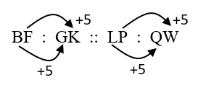Question 178
If the BLOOD is coded as 24113 and BRUST as 20678, then code for ROBUST is :
 A 620781 B 612078 C 678102 D 610732
Mathematical-Reasoning       Coding-and-Decoding       UGC NET Dec 2004 paper-1
Question 178 Explanation:Question 179
A bag contains an equal number of one rupee, 50 paise and 25 paise coins. If the total amount in the bag is Rs. 35, how many coins of each type are there ?
 A 15 B 18 C 20 D 25
Mathematical-Reasoning       Ratio-and-Proportion       UGC NET Dec 2004 paper-1
Question 179 Explanation:
If consider equal number of coins = 20
Then 20 ×1=20
20×0.5=10
20×0.25=5
Total = 35
 Question 180
In the sequence of numbers (⅔), (4/7), X, 11/21, 16/31 the missing number X is :
 A 8/10 B 6/10 C 5/10 D 7/10
Mathematical-Reasoning       Series-Test       UGC NET Dec 2004 paper-1
Question 180 Explanation:Question 181
If A stands for 5 , B for 6, C for 7, D for 8, and so on, what do the following numbers stand for : 22, 25, 8, 22 and 5 ?
 A PRIYA B NEEMA C MEENA D RUDRA
Mathematical-Reasoning       Coding-and-Decoding       UGC NET Dec 2004 paper-1
Question 181 Explanation:
A = 5, B = 6, C = 7
A = (1+4), B = (2+4), C = (3+4) ……….
22, 25, 8, 22, 5 = (18+4), (21+4), (4+4), (8+4), (1+4)
= RUDRA
 Question 182
Which of the following statements say the same thing ?
(a) “I am clever” (said by Rama)
(b) ”I am clever” (said by Raju)
(c) “My son is clever” (said by Rama’s father)
(d) ”My brother is clever” (said by Rama’s sister)
(e) ”My brother is clever” (said by Rama’s only sister)
(f) ”My sole enemy is clever” (said by Rama’s only enemy)
Choose the correct answer from the code given below :
 A (a), (c), (d), (e) and (f) B (a) and (b) C (d) and (e) D (a) and (f)
Mathematical-Reasoning       Blood-Relation-Test       UGC NET Dec 2004 paper-1
Question 182 Explanation:
Statements a, c, d, e, f are giving the same thing i.e., about Rama.
 Question 183
The number of students in two classes A and B and the respective mean of the marks obtained by each of the class are given in the following table : Class A Class B Number of Students 20 80 Arithmatic Mean 10 20 The combined mean of the marks of the two classes will be :
 A 18 B 15 C 10 D 20
Mathematical-Reasoning       Averages       UGC NET Dec 2004 paper-1
 Question 184
The next term in the series −1, 5, 15, 29, ? , ... is :
 A 36 B 47 C 59 D 63
Mathematical-Reasoning       Series-Test       UGC-NET CS 2018 JUNE Paper-1
Question 184 Explanation:
It can be observed that the difference between two consecutive terms is increasing by adding 4
in the previous difference.
So for next term, next difference will be 14+4= 18
Next term = 29 + 18
Next term = 47
 Question 185
The next term in the series
ABD, DGK, HMS, MTB, SBL, ? , ... is :
 A ZKU B ZCA C ZKW D KZU
Mathematical-Reasoning       Alphabet-Test       UGC-NET CS 2018 JUNE Paper-1
Question 185 Explanation:Question 186
If VARANASI is coded as WCUESGZQ, then the code of KOLKATA will be :
 A LOQOZEH B HLZEOOQ C ZELHOQO D LQOOFZH
Mathematical-Reasoning       Coding-and-Decoding       UGC-NET CS 2018 JUNE Paper-1
Question 186 Explanation:Question 187
Introducing Rakesh to her husband a women said, “His brother’s father is the only son of my grandfather”. The woman is related to Rakesh as :
 A Aunt B Mother C Sister D Daughter
Mathematical-Reasoning       Blood-Relation-Test       UGC-NET CS 2018 JUNE Paper-1
Question 187 Explanation:Question 188
Two numbers are in the ratio 2 : 5. If 16 is added to both the numbers, their ratio becomes 1 : 2. The numbers are :
 A 16, 40 B 20, 50 C 28, 70 D 32, 80
Mathematical-Reasoning       Ratio-and-Proportion       UGC-NET CS 2018 JUNE Paper-1
Question 188 Explanation:
x/y = 2/5
⇒ x = (2 /5 )y ------(1)
(x+16)/(y+16)= 1/ 2
2 x + 3 2 = y + 1 6
Put value of ′ x ′ from eqn.(1) into above eqn.
2 ( (2 /5) y ) + 3 2 = y + 1 6
4 y + 160 = 5 y + 8 0
y = 80
Put value of ′ y ′ in eqn.(1)
x = (2/5) × 80
x = 3 2
 Question 189
Which of these words is different from the rest ?
 A Sharp B Tail C Thin D Huge
Mathematical-Reasoning       Analogy-Test       UGC NET CS 2018 DEC Paper-1
 Question 190
​ If FACE is coded as HCEG, then the code for HIGH will be :
 A JKIJ B ZXYZ C BEFB D KHIK
Mathematical-Reasoning       Coding-and-Decoding       UGC NET CS 2018 DEC Paper-1
Question 190 Explanation:Question 191
The next term in the letter series DY, JX, OW, SV, VU, ______ is :
 A XS B XT C WV D YT
Mathematical-Reasoning       Alphabet-Test       UGC NET CS 2018 DEC Paper-1
Question 191 Explanation:Question 192
The next number in the series 12, 15, 21, 33, 57, ______, is :
 A 97 B 107 C 105 D 95
Mathematical-Reasoning       Series-Test       UGC NET CS 2018 DEC Paper-1
Question 192 Explanation:
Here the next difference = 2* previous difference.
So next term = previous term+ next difference.
next term = 57+ 48 = 105
 Question 193
Ram said to Shyam “That girl playing with the doll, is the younger of the two daughters of my father’s wife” .
How the girl playing with the doll is related to Ram ?
 A Sister-in-law B Cousin C Aunty D Sister
Mathematical-Reasoning       Blood-Relation-Test       UGC NET CS 2018 DEC Paper-1
Question 193 Explanation:Question 194
In a new budget, the price of petrol rose by 25%, By how much percent must a person reduce his consumption so that his expenditure on it does not increase?
 A 10% B 15% C 20% D 25%
Mathematical-Reasoning       Percentage       UGC-NET June CS 2019 Paper 1
Question 194 Explanation:
Let the price of the petrol is 100
It’s prices is increased by 25% then new price is 125.
Let Percentage of decrease of consumption =X
Then 125*X /100=25
⇒ X=2500/125
⇒ 20%
 Question 195
For all integers y>1, (y)=2y+(2y-1)+(2y-2)+..+1. What is the value of (3)x(2)? Where x is a multiplication operator?
 A 116 B 210 C 263 D 478
Mathematical-Reasoning       Number-Theory       UGC-NET June CS 2019 Paper 1
 Question 196
A sum of money doubles at compound interest in 6 years. In how many years will it become 16 times?
 A 16 years B 24 years C 48 years D 96 years
Mathematical-Reasoning       Compound-Interest       UGC-NET June CS 2019 Paper 1
Question 196 Explanation:
Assume the initial sum is x,
After six years, the amount becomes double which is 2x
After 12 years, again the amount is double(compound interest), which is 4x
After 18 years, again the amount is double(compound interest), which is 8x
After 24 years,again the amount is double(compound interest), which is 16x
 Question 197
Oar is to rowboat as foot is to
 A running B sneaker C skateboard D jumping
Mathematical-Reasoning       Analogy-Test       UGC-NET June CS 2019 Paper 1
Question 197 Explanation:
An oar puts a rowboat into motion.
A foot puts a skateboard into motion.
The answer is not choice (a), because running is not an object that is put into motion by a foot.
Sneaker (choice b) is incorrect because it is something worn on a foot.
Jumping (choice d) is incorrect because although you do need feet to jump, jumping is not an object that is put into motion by means of a foot.
 Question 198
If 152 is divided into four parts proportional to 3,4,5 and 7, then the smallest part is
 A 29 B 26 C 25 D 24
Mathematical-Reasoning       Number-Theory       UGC-NET June CS 2019 Paper 1
Question 198 Explanation:
Given four proportional are 3x,4x,5x,7x.
Four proportional are divided into 152.
3x+4x+5x+7x=152
19x=152⇒ x=8
The smallest proportional is 3x=3x8=24
 Question 199

A certain principal invested at compounded interest payable yearly amounts to Rs. 10816.00 in 3 years and Rs. 11248.64 in 4 years. What is the rate of interest?

 A 3% B 4% C 4.5% D 5.5%
Mathematical-Reasoning       Compound-Interest       UGC-NET Dec 2019 Part-1
Question 199 Explanation:
Rate of interest=((11248.64 - 10816)/10816) * 100
= (432.64 / 10816)*100
= 4%
 Question 200

In a certain coding language, ‘AEIOU’ is writing as ‘TNHDZ’. Using the same coding language, ‘BFJPV’ will be written as

 A UOIEA B AEIOUS C CGKQW D WQKGC
Mathematical-Reasoning       Coding-and-Decoding       UGC-NET Dec 2019 Part-1
Question 200 Explanation:
'AEIOU' is written as 'TNHDZ'
The above code following 2 conditions
1. character-1
2. Firs position is replaced with last position
A-1= Z and placed in last
E-1= D placed in second last
I-1=H placed in third last
O-1=N placed in fourth last
S-1=T placed in first position

The same order will follow for 'BFJPV'
B-1= A placed in last
F-1=E is placed in second last
J-1=I is placed in third last
P-1=O is placed in fourth last
V-1=U is placed in first position.
 Question 201

What is the exact equivalent discount of three successive discounts of 10%, 15% and 20% by sale of an article?

 A 35.8% B 38.5% C 35.5% D 38.8%
Mathematical-Reasoning       Profit-and-Loss       UGC-NET Dec 2019 Part-1
Question 201 Explanation:
Single equivalent discount for successive discounts of 10% and 15%.
= (10+15- (15*10)/100)%
= 23.5%
Single equivalent discount for 23.5% and 20%
=(23.5+20-(23.5*20)/100)%
=38.8%
 Question 202

If 3% of (a + b) = 7% of (ab) and 5% of (a – b) = 4% of (ab) then what percentage of b is a?

 A 47/23 % B 23/47 % C 4700/23 % D 2300/47 %
Mathematical-Reasoning       Percentage       UGC-NET Dec 2019 Part-1
 Question 203

The sum of a number and its inverse is –4. The sum of their cubes is:

 A -52 B 52 C 64 D -64
Mathematical-Reasoning       Number-Theory       UGC-NET Dec 2019 Part-1
Question 203 Explanation:
Given : a + 1/a = - 4
Find: a^3 + (1/a)^3
Solution: a + 1/a = -4
Take cube on both sides
(a + 1/a)^3 = (-4)^3
a^3 + (1/a)^3 + 3*a* 1/a (a+1/a) = -64
a^3 + (1/a)^3 + 3(a+1/a) = -64
a^3 + (1/a)^3 - 12 = -64
a^3 + (1/a)^3 = - 64 + 12
a^3 + (1/a)^3 = -52
There are 203 questions to complete.